##### The Japanese Bonsai specialist
Direct order Contact Help / Services Newsletter# Adjustable chrome-plated vise 130 mm

Tord tronc 130 mm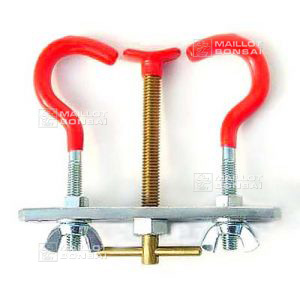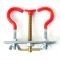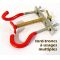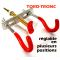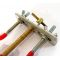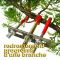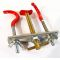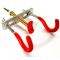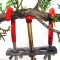ref. : 4329

23,00

Available quantity : 8Order

###### Description

Width 130 mm. To give a curl to a trunk or large branches. In galvanized metal with plastic protection.

#adjustable 3 #chrome 2.6 #bonsai 2.6 #plated 2.6 #tools 2.5 #vise 2.4 #galvanized 2 #protection 2 #branches 1.8 #plastic 1.7

Formule
(( ROUND((CHAR_LENGTH(b.article_nom)-CHAR_LENGTH(REPLACE(b.article_nom, 'adjustable', '')))/LENGTH('adjustable')) + ROUND((CHAR_LENGTH(b.article_description)-CHAR_LENGTH(REPLACE(b.article_description, 'adjustable', '')))/LENGTH('adjustable')) ) * 3) + (( ROUND((CHAR_LENGTH(b.article_nom)-CHAR_LENGTH(REPLACE(b.article_nom, 'chrome', '')))/LENGTH('chrome')) + ROUND((CHAR_LENGTH(b.article_description)-CHAR_LENGTH(REPLACE(b.article_description, 'chrome', '')))/LENGTH('chrome')) ) * 2.6) + (( ROUND((CHAR_LENGTH(b.article_nom)-CHAR_LENGTH(REPLACE(b.article_nom, 'plated', '')))/LENGTH('plated')) + ROUND((CHAR_LENGTH(b.article_description)-CHAR_LENGTH(REPLACE(b.article_description, 'plated', '')))/LENGTH('plated')) ) * 2.6) + (( ROUND((CHAR_LENGTH(b.article_nom)-CHAR_LENGTH(REPLACE(b.article_nom, 'vise', '')))/LENGTH('vise')) + ROUND((CHAR_LENGTH(b.article_description)-CHAR_LENGTH(REPLACE(b.article_description, 'vise', '')))/LENGTH('vise')) ) * 2.4) + (( ROUND((CHAR_LENGTH(b.article_nom)-CHAR_LENGTH(REPLACE(b.article_nom, 'galvanized', '')))/LENGTH('galvanized')) + ROUND((CHAR_LENGTH(b.article_description)-CHAR_LENGTH(REPLACE(b.article_description, 'galvanized', '')))/LENGTH('galvanized')) ) * 2) + (( ROUND((CHAR_LENGTH(b.article_nom)-CHAR_LENGTH(REPLACE(b.article_nom, 'protection', '')))/LENGTH('protection')) + ROUND((CHAR_LENGTH(b.article_description)-CHAR_LENGTH(REPLACE(b.article_description, 'protection', '')))/LENGTH('protection')) ) * 2) + (( ROUND((CHAR_LENGTH(b.article_nom)-CHAR_LENGTH(REPLACE(b.article_nom, 'branches', '')))/LENGTH('branches')) + ROUND((CHAR_LENGTH(b.article_description)-CHAR_LENGTH(REPLACE(b.article_description, 'branches', '')))/LENGTH('branches')) ) * 1.8) + (( ROUND((CHAR_LENGTH(b.article_nom)-CHAR_LENGTH(REPLACE(b.article_nom, 'plastic', '')))/LENGTH('plastic')) + ROUND((CHAR_LENGTH(b.article_description)-CHAR_LENGTH(REPLACE(b.article_description, 'plastic', '')))/LENGTH('plastic')) ) * 1.7) + (( ROUND((CHAR_LENGTH(b.article_nom)-CHAR_LENGTH(REPLACE(b.article_nom, 'large', '')))/LENGTH('large')) + ROUND((CHAR_LENGTH(b.article_description)-CHAR_LENGTH(REPLACE(b.article_description, 'large', '')))/LENGTH('large')) ) * 1.5) + (( ROUND((CHAR_LENGTH(b.article_nom)-CHAR_LENGTH(REPLACE(b.article_nom, 'metal', '')))/LENGTH('metal')) + ROUND((CHAR_LENGTH(b.article_description)-CHAR_LENGTH(REPLACE(b.article_description, 'metal', '')))/LENGTH('metal')) ) * 1.5)

## Secure payment## Delivery

Our logistic partners :04 74 55 23 48
Pépinière MAILLOT-BONSAÏ
Le Bois Frazy
01990 RELEVANT - FRANCE
on appointment